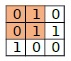# Check if matrix A can be converted to B by changing parity of corner elements of any submatrix in Python

Suppose we have two N X M binary matrices A and B. In a single operation, we can select a sub-matrix (at least 2x2) and convert the parity of the corner elements (flip bits). Finally, we have to check whether the matrix A can be converted to B by performing any number of operations or not.

So, if the input is like

 1 0 0 1 0 1 1 0 0then the output will be True as we can perform the operation on the top left square sub-matrix of size (2x2) on mat1 to get mat2.

To solve this, we will follow these steps −

• row := row count of mat1
• column := column count of mat1
• for i in range 1 to row - 1, do
• for j in range 1 to column - 1, do
• if mat1[i, j] is not same as mat2[i, j], then
• mat1[i, j] := mat1[i, j] XOR 1
• mat1[0, 0] := mat1[0, 0] XOR 1
• mat1[0, j] := mat1[0, j] XOR 1
• mat1[i, 0] := mat1[i, 0] XOR 1
• for i in range 0 to row - 1, do
• for j in range 0 to column - 1, do
• if mat1[i, j] is not same as mat2[i, j], then
• return False
• return True

## Example

Let us see the following implementation to get better understanding −

Live Demo

def solve(mat1, mat2):
row = len(mat1)
column = len(mat1)
for i in range(1, row):
for j in range(1, column):
if mat1[i][j] != mat2[i][j]:
mat1[i][j] ^= 1
mat1 ^= 1
mat1[j] ^= 1
mat1[i] ^= 1
for i in range(row):
for j in range(column):
if mat1[i][j] != mat2[i][j]:
return False
return True
mat1 = [
[1, 0, 0],
[1, 0, 1],
[1, 0, 0]]
mat2 = [
[0, 1, 0],
[0, 1, 1],
[1, 0, 0]]
print(solve(mat1, mat2))

## Input

[
[1, 0, 0],
[1, 0, 1],
[1, 0, 0]],
[
[0, 1, 0],
[0, 1, 1],
[1, 0, 0]]

## Output

True